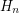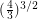# Counting 3-colorings of the hex lattice

 Importance: Medium ✭✭
 Author(s): Thomassen, Carsten
 Subject: Graph Theory » Coloring » » Vertex coloring
 Keywords: coloring Lieb's Ice Constant tiling torus
 Posted by: mdevos on: July 5th, 2008
Problem   Find.

We'll begin by putting in place the necessary notation. Letbe the regular triangular tiling of the plane. For everythere is a regular map which triangulates the torus, denoted, which may be obtained from a regular hexagonal piece ofof side-lengthby identifying points on opposite edges of this hexagon. Letbe the dual of(on the torus). Thenis a regular map on the torus - a hexagonal tiling. One last definition: for any graphand any positive integerwe letdenote the number of proper-coloring of.

A famous theorem of Lieb [L] shows thatwheredenotes thequadrangulation of the torus. This theorem is usually stated in terms of Eulerian orientations, and is of interest to physicists as the constant(called Lieb's Ice Constant) determines the "residual entropy for square ice".

Thomassen proved that every planar graphwith girthhas exponentially many proper 3-colorings. More precisely, he showed that. This gives a lower bound on the limit in the above problem (assuming it exists).

## Bibliography

[L] E. H. Lieb, Exact Solution of the Problem of the Entropy of Two-Dimensional Ice. Phys. Rev. Lett. 18, 692-694, 1967.

* indicates original appearance(s) of problem.